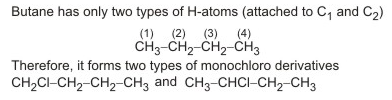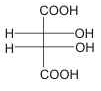Courses

# VITEEE Chemistry Test - 9

## 40 Questions MCQ Test VITEEE: Subject Wise and Full Length MOCK Tests | VITEEE Chemistry Test - 9

Description
This mock test of VITEEE Chemistry Test - 9 for JEE helps you for every JEE entrance exam. This contains 40 Multiple Choice Questions for JEE VITEEE Chemistry Test - 9 (mcq) to study with solutions a complete question bank. The solved questions answers in this VITEEE Chemistry Test - 9 quiz give you a good mix of easy questions and tough questions. JEE students definitely take this VITEEE Chemistry Test - 9 exercise for a better result in the exam. You can find other VITEEE Chemistry Test - 9 extra questions, long questions & short questions for JEE on EduRev as well by searching above.
QUESTION: 1

Solution:
QUESTION: 2

Solution:
QUESTION: 3

### Acetaldehyde and acetone differ in their reaction with

Solution:
QUESTION: 4

Carbonyl compounds undergo nucleophilic addition because of

Solution:
QUESTION: 5

Acid catalysed hydration of alkenes except ethene leads to the formation of

Solution:
QUESTION: 6

Which of the following is not a unit of time?

Solution:
QUESTION: 7

Irrespective of the source, pure sample of water always yields 88.89% mass of oxygen and 11.11% mass of hydrogen. This is explained by the law of

Solution:
QUESTION: 8

Which compound can exist in a dipolare (Zwitterion) structure?

Solution:
QUESTION: 9

An organic compound with the formula of C₆H₁₂O₆ forms a yellow crystalline solid with phenylhydrazine and gives a mixture of sorbitol and mannitol when reduced with sodium. Which among the following could be the compound?

Solution:
QUESTION: 10

The tripeptide hormone present in most living cells is

Solution:
QUESTION: 11

How many monochlorobutanes will be obtained on chlorination of n-butane?

Solution:QUESTION: 12

Saponification of ethyl benzoate with caustic soda as alkali, gives

Solution:
QUESTION: 13

Which salt can be produced by the reaction of carbon monoxide and caustic soda (NaOH)?

Solution:
QUESTION: 14

According to Le-Chatelier's principle, adding heat to a solid and liquid in equilibrium will cause the

Solution:
QUESTION: 15

Which of the following does not contain a carboxyl group?

Solution:
QUESTION: 16

A vessel at equilibrium, contains SO₃, SO₂ and O₂. Now some helium gas is added, so that total pressure increases while temperature and volume remain constant. According to Le-Chatelier's principle, the dissociation of SO₃

Solution:
QUESTION: 17

Consider the following reaction occuring in a automobile engine 2C₈H₈(g) + 25O₂(g) → 16CO₂(g) + 18H₂O(g). The signs of ∆H, ∆S and ∆G for above reaction would be

Solution:
QUESTION: 18

An endothermic reaction is one in which

Solution:
QUESTION: 19

The difference between ΔH and ΔE for the combustion of methane at 270C will be (in J mol-1)

Solution:

CH4(g) + 2O2 → CO2(g) + 2H2O(l)
Δn = 1 - 3 = (-2)
∴ ΔH - ΔE = Δng RT = (-2) (8.314) (300)

QUESTION: 20

Which of the following is an azo dye?

Solution:
QUESTION: 21

CH₃CH₂CH₂CH(CH=CH₂)CH₂CH₂CH₃ is

Solution:
QUESTION: 22

Which of the following complex exhibits the highest paramagnetic behaviour?
(where gly = glycine, en = ethylene diamine and bpy = bipyridyl moities (At Nos. Ti = 22, V = 23, Fe = 26, Co = 27)

Solution:
QUESTION: 23

Sodium nitroprusside reacts with sulphide ion to give a purple colour due to the formation of :

Solution:
QUESTION: 24

Alkyl cyanides are

Solution:
QUESTION: 25

Which of the following compound gives dye test ?

Solution:
QUESTION: 26

When the same quantity of electricity is passed through the solution of different electrolytes in series, the amounts of product obtained are proportional to their

Solution:
QUESTION: 27

In which of the following solutions are ions present?

Solution:
QUESTION: 28

A cell constant is generally found by measuring the conductivity of aqueous solution of

Solution:
QUESTION: 29

The reagent used for the preparation of higher ethers from halogenated ethers is

Solution:
QUESTION: 30

If the melting point of the metals are lower than those of impurities, then the crude metals are purified by the process of

Solution:
QUESTION: 31

The relationship which describes the variation of vapour pressure with temperature is called

Solution:
QUESTION: 32

Which of the following is not acidic?

Solution:
QUESTION: 33

The element with the highest first ionization potential is

Solution:
QUESTION: 34

Phosphine is produced by adding water to

Solution:
QUESTION: 35

In CsCl structure, each Cs⁺ ion is surrounded by

Solution:
QUESTION: 36

Consider the following reaction, 5H₂O₂ + ClO₂ + 2OH- → x Cl- + y O₂ + 6H₂O The reaction is balanced if

Solution:
QUESTION: 37

Following stereo-structure of tartaric acid represents:Solution:
QUESTION: 38

Which of the following type of molecules from micelles?

Solution:
QUESTION: 39

Cuprous ion is colourless while cupric ion is coloured because

Solution:
QUESTION: 40

The formula of haematite is

Solution: